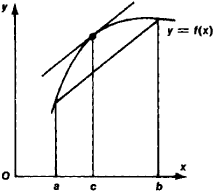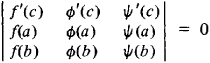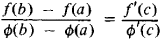# Mean Value Theorem

(redirected from Cauchy's mean value theorem)

## mean value theorem

[′mēn ′val·yü ‚thir·əm]
(mathematics)
The proposition that, if a function ƒ (x) is continuous on the closed interval [a,b ] and differentiable on the open interval (a,b), then there exists x0, a <>x0<>b, such that ƒ(b) - ƒ(a) = (b-a)ƒ′(x0). Also known as first law of the mean; Lagrange's formula; law of the mean.
McGraw-Hill Dictionary of Scientific & Technical Terms, 6E, Copyright © 2003 by The McGraw-Hill Companies, Inc.
The following article is from The Great Soviet Encyclopedia (1979). It might be outdated or ideologically biased.

## Mean Value Theorem

one of the fundamental results of the differential calculus relating an increment of a function f(x) and the values of its derivative. In analytic terms,

f(b) — f(a) = (b — a)f’(c)

where c is some number satisfying the inequality a < c < b. Formula (1) is valid if the function f(x) is continuous on the segment [a, b] and has a derivative at each point of the interval (a, b). In geometric terms (see Figure 1), formula (1) states that the tangent to the curve y = f(x) at a suitable point [c, f(c)] is parallel to the chord passing through the points [a, f(a)] and [b, f(b)]. The mean value theorem was discovered by J. Lagrange in 1797.Figure 1

Among the different generalizations of the mean value theorem, note Bonnet’s mean value formulaand its particular case, Cauchy’s mean value formula